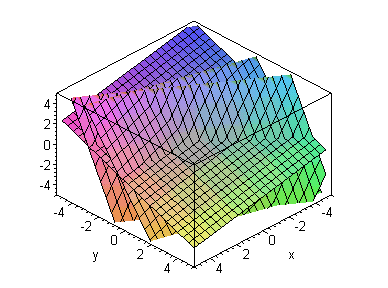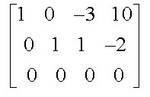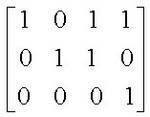## Friday, 13 March 2009

### Planes in Space - Part twoConsistent solutions to three planes

Now let’s add to our system from the last blog and make a system of three equations; {2x + 3y – 3z = 14 , –3x + y + 10z = -32, x + 7y + 4z = -4}. If we try to solve this system by the inverse method we get the dreaded “Singular Mat” error on the calculator and all we can determine is that there is NOT a single unique solution. If we use the RREF we get something that should remind us of our previous example, and for good reason. Because I chose the third line to be a linear combination of the first two, the three planes all share a common line of intersection, the line (x, y, z) = (10, -2, 0) + t [3, -1, 1] as shown by the reduced form:The third row gives us an obviously true statement (0x + 0y + 0z = 0) that reflects that the third equation, in essence, added no new information to the system. This “row of zeros” at the bottom is the key to the student that the system had three equations but the intersection would be completely determined by any two of them.

When three planes intersect in space, there are six basic configurations (They sometimes miss the sixth possible case that all three equations are for the same plane) . I ask students to visualize and describe the different situations that can exist prior to discussion of the method of finding the solutions. In most years they come up with all five configurations and names for them. Here is a list of the five/six as my students tend to describe them.

The two consistent systems are three planes intersecting in a single point, and three planes intersecting in a single line (the book binding model – the planes are pages in a book and the common line is the binding).

The three configurations with no common points of intersection are three parallel planes, two parallel planes cut by a transverse plane (the H configuration) forming two parallel lines, and three planes intersecting in mutual pairs to form three parallel lines (the delta D configuration). The first and the second are often easy to recognize from the equations since the parallels are usually recognizable, but the third is difficult to identify without reduction.

Three configurations w/o a common solution
If we look at the three inconsistent systems possible with three planes, the more obvious cases may lead us to more understanding of the less obvious cases. First I want to look at three parallel planes, x + 2y + 3z = 1, x+ 2y + 3z = 5, and 2x + 4y + 6z = 10.
The reduced RREF augmented matrix looks like this:The first row looks like it is directly related to the coefficients of x, y, and z in each equation, but the 0 in the constant term may seem perplexing. I think of this information as a direction vector [1,2,3] that is perpendicular to the three parallel planes. The bottom line of zeros reminds us that the last equation added no new information about the solution set. But what are we to make of the equation for the second row, 0x + 0y + 0z = 1. I tell students that this is the signal that there is NO common point of intersection. In essence, we have an intersection when 0=1, and that is never true. If the three equations had all represented the same plane, then the original line would have contained the constant term as well [1, 2, 3, 1], and is in essence, just a version of the original plane.

Now we examine a system with two of the same parallels and a third plane not parallel to them, the H configuration, { x + 2y + 3z = 1, x+ 2y + 3z = 5, 2x – y + z = 1}. The reduced matrix looks like .How can we interpret this. The bottom row is our old insolvable friend 0x +0y + 0z = 1, reminding us that there can be no common point on the three planes (since two of them are parallel). The upper two rows look like the rows when we had a line of intersection except that both constant terms equal zero. Does this tell us anything about the two lines of intersection formed by the plane cutting through the two parallel planes. Perhaps we can find out more if we find the two intersections one at a time. If we reduce the two equations x + 2y + 3z = 3, 2x – y + z = 1, our reduced matrix looks like this,This can be read as the system of equations x+z=1 and y+z=1... so setting z = 0 first we get a point (1,1,0) on the intersection, and using z=1 we get (0, 0, 1)

. This gives a line (x, y, z) = (1, 1, 0) + t 1, 1, -1] . If we do the intersection with the second parallel plane we get (x, y, z) = (1.4, 1.8, 0) + t [1, 1, -1]. It seems that our original reduced system was flashing the direction vector part of the two parallel lines created by the three planes in the third column.

For our final case, we pick the inconsistent situation which is most difficult to distinguish from the consistent cases, the delta D configuration in which three planes intersect in mutual pairs to form three parallel lines. An example is the three planes defined by { x + y + 7z = 5, x – y – z = 3, and 2x – y + 2z = 4} .

The reduced form looks like this,and we are now becoming accustom to the 0001 on the bottom reminding us there is no common point for the three planes. And a quick inspection of the three planes assures us that no two of them are parallel, so the only possible case is the “delta formation” and three parallel lines of mutual intersection. What are we to make of the two upper rows. Our experience now leads us to think that [3, 4,-1] will be the direction vector for all three lines. We can verify our supposition by taking the vectors in pairs and reducing the two-equation systems to find the actual line of intersection, but as is the fashion in mathematical writing, I leave that final task as an exercise for the reader.